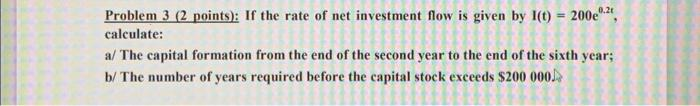# Question Problem 3 (2 points): If the rate of net investment flow is given by I(t) = 2002, calculate: a/The capital formation from the end of the second year to the end of the sixth year; b/The number of years required before the capital stock exceeds $200 000DMK0T4 The Asker · Advanced MathematicsTranscribed Image Text: Problem 3 (2 points): If the rate of net investment flow is given by I(t) = 2002, calculate: a/The capital formation from the end of the second year to the end of the sixth year; b/The number of years required before the capital stock exceeds$200 000
More
Transcribed Image Text: Problem 3 (2 points): If the rate of net investment flow is given by I(t) = 2002, calculate: a/The capital formation from the end of the second year to the end of the sixth year; b/The number of years required before the capital stock exceeds \$200 000